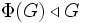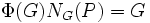# Frattini subgroup is nilpotent in finite

This article gives the statement, and possibly proof, of the fact that for any Finite group (?), the subgroup obtained by applying a given subgroup-defining function always satisfies a particular subgroup property
View all such group property satisfactions OR View more information on subgroup-defining functions in finite groups
This article describes a fact or result that is not basic but it still well-established and standard. The fact may involve terms that are themselves non-basic
View other semi-basic facts in group theory
VIEW FACTS USING THIS: directly | directly or indirectly, upto two steps | directly or indirectly, upto three steps|

## Statement

### Verbal statement

The Frattini subgroup of any finite group is nilpotent.

### Symbolic statement

Let$G$ be a finite group and$\Phi(G)$ denote the intersection of all maximal subgroups of$G$ (the so-called Frattini subgroup of$G$).

## Definitions used

### Frattini subgroup

Further information: Frattini subgroup

The Frattini subgroup of a (here, finite) group is the intersection of all its maximal subgroups.

### Finite nilpotent group

Further information: Finite nilpotent group A finite group is nilpotent if every Sylow subgroup of it is normal.

## Generalizations

The result generalizes in two important respects. First, we can prove considerably stronger results about Frattini subgroups for finite groups, and more generally, for groups in which every proper subgroup is contained in a maximal subgroup. Second, we can generalize to proving the results about any Frattini-embedded normal subgroup of an arbitrary group.

For instance:

## Proof

Given: A finite group$G$, and$\Phi(G)$ is the Frattini subgroup

To prove: For any Sylow subgroup$P$ of$\Phi(G)$,$P$ is normal in$\Phi(G)$.

Proof: In fact, we shall show that$P$ is normal in$G$.

Here's the idea. By applying Frattini's argument and the fact that$\Phi(G) \triangleleft G$, we have$\Phi(G)N_G(P) = G$. Now if$N_G(P) \ne G$, it is contained in a maximal subgroup$M$ of$G$. Since$\Phi(G)$ is contained in every maximal subgroup,$\Phi(G)N_G(P) \le M$, leading to a contradiction.